（2018年5月23日，广州）一年一度的腾讯“云+未来”峰会在广州隆重召开。作为腾讯年度最大型活动之一，“云+未来”峰会承载了腾讯产业生态的宏大愿景，更是腾讯各业务条线及合作伙伴炫技的舞台。以英特尔、甲骨文、中国银行、广汽集团等为代表的世界500强，以及近期炙手可热的VIPKID、创梦天地、永辉云创等明星企业，纷纷亮相峰会的舞台与展台。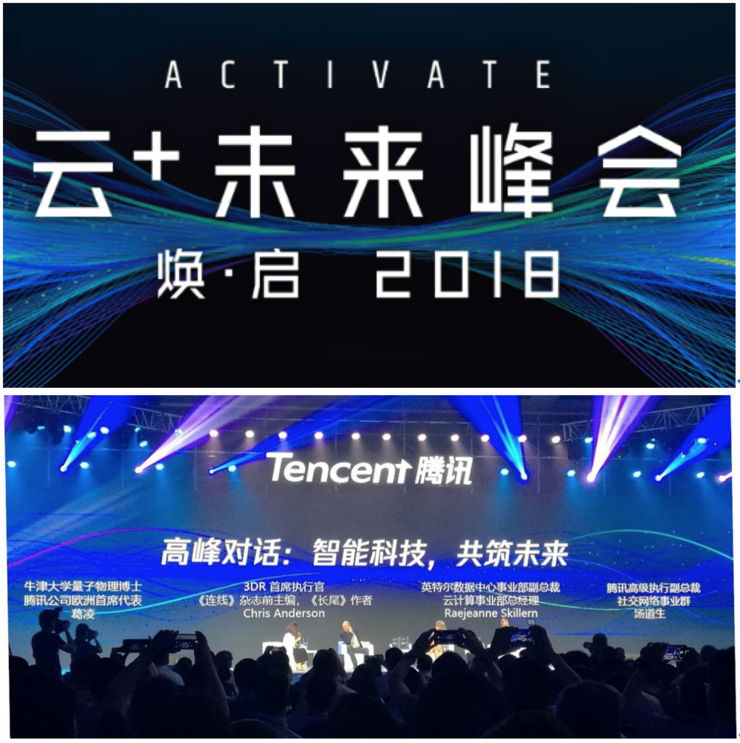作为本次峰会的特别合作伙伴，腾讯音乐娱乐集团旗下专注企业服务的成员公司爱听卓乐出席了峰会，向来自国内外数以千计的观众展示了“爱听车乐云”——专门针对汽车客户量身定做的音乐服务解决方案。 爱听卓乐在展台上展示了搭载有“爱听车乐云”系统的汽车和车机，以其海量正版的优质曲库、紧贴流行的歌单榜单、知情识趣的个性化推荐、以及智能化的人机交互，刷新了观众对数字时代车载娱乐服务的认知。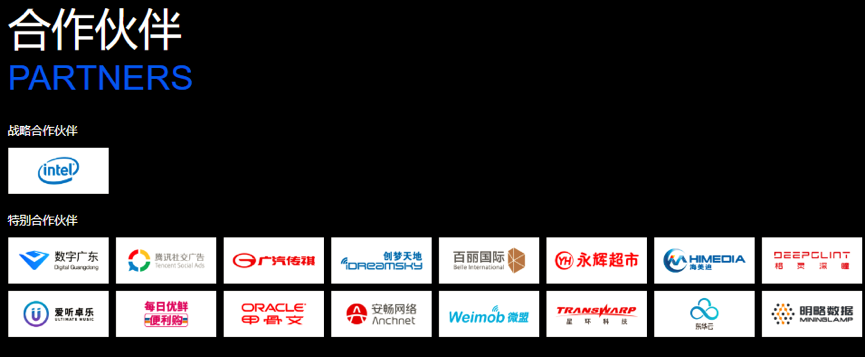繁忙的爱听卓乐展台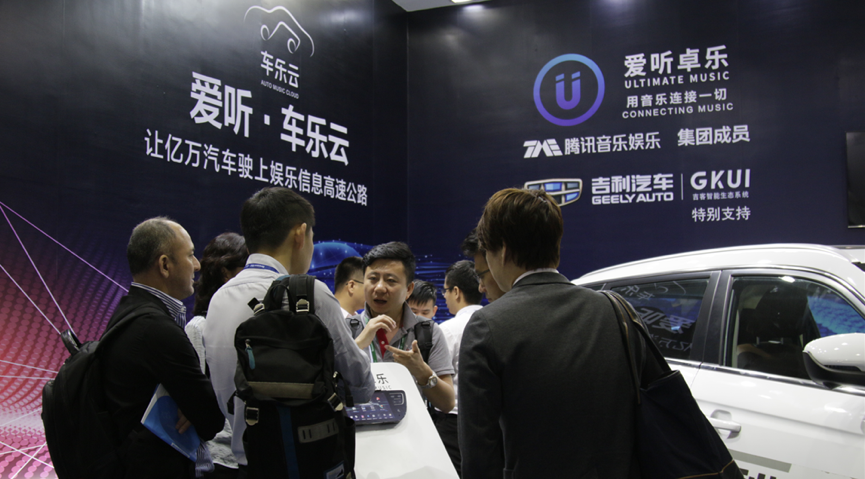海外客户对“爱听车乐云”解决方案兴趣盎然腾讯音乐娱乐集团企业服务副总裁、爱听卓乐联合创始人丁纲在大会期间接受了媒体的采访，分享了爱听卓乐对于车载娱乐服务、智能交互体验等方面的深入思考和独特见解：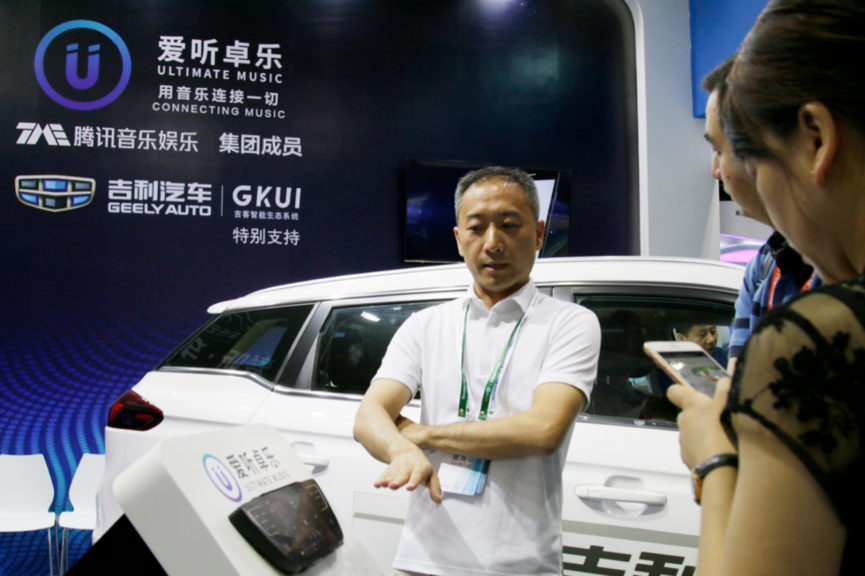腾讯音乐娱乐集团企业服务副总裁、爱听卓乐联合创始人丁纲

丁纲：用音乐服务打开汽车用户运营之门

传统企业与互联网公司的最大区别之一是，前者比较关注如何把产品卖出去，后者则更关注用户的获取和运营。

正如手机不再仅仅用于打电话，汽车也从一个代步工具进化成为一个“如影随形的智能化移动终端”。随着AI技术和车联网的发展，汽车正变得越来越“手机化”。不管是传统车企还是新锐造车势力，都纷纷聚焦如何优化人机交互、提升用户体验，探讨何种服务最能与用户产生更紧密、更频繁、更持久的连接，从而打开用户全生命周期运营的大门。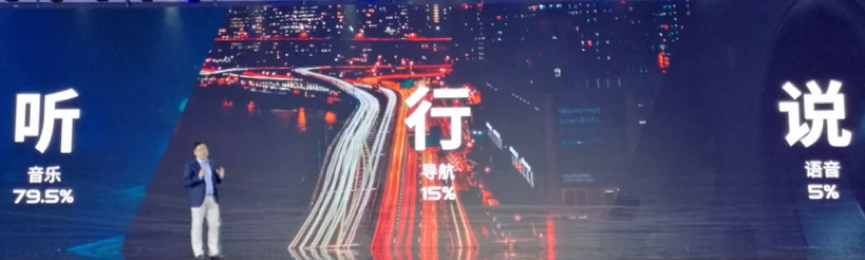调研数据显示，在众多的车载服务当中，使用时长和流量消耗占比最高的是音乐，比重高达80%，甚至远远超过了导航（占比仅为15%）。由此可见，在车内提供优质的音乐服务、满足用户的核心刚需，是进行汽车用户运营的重中之重，可以起到四两拨千斤的效果。

丁纲：内容平台的开放性带来的用户交互体验的提升，是车载音乐服务的决胜关键

车载音乐服务成功的关键，除了曲库质量，更重要的是用户交互的智能化体验，而音乐服务平台的开放性，能够最大程度地提升用户交互的智能化：有别于其他的内容服务商，爱听卓乐坚持内容平台的开放性，在合作中能够与语音方案商及汽车企业进行更深层的融合和更底层的对接，分享包括各种内容标签在内的曲库数据，为AI语音识别创造更好的训练条件，从而实现场景化和个性化的推荐，带来更智能化的人机交互、更良好的用户体验。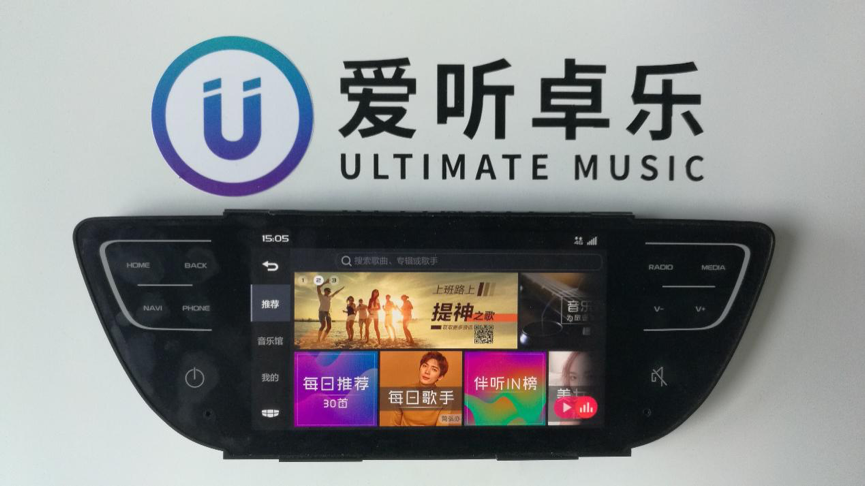丁纲：对于有志打造自有品牌音乐应用的车企，爱听卓乐甘当其幕后推手

有别于绝大多数的内容服务提供商，爱听卓乐是数字音乐领域屈指可数的专注服务B端的企业，在用户获取方面与车企不存在利益分割和冲突，甘于隐身幕后提供专业专注的企业级服务，输出源源不断的优质内容，帮助车企打造自主品牌的音乐应用、获取并运营完全属于自己的用户。

对于整车厂商来说，车内娱乐场景的提升渐渐成为品牌竞争力的一部分，打造自有品牌的车载娱乐系统被越来越多车企视为构建具备品牌特色的服务体验、维系用户忠诚度的重要手段。有见及此，爱听卓乐专门整合推出了“爱听·车乐云”车载音乐服务解决方案，向车企输出优质的内容资源、定制化的技术方案、个性化的内容运营，大幅降低了车厂自主打造音乐服务的门槛，让车厂能以更高的性价比，实现用户体验的提升。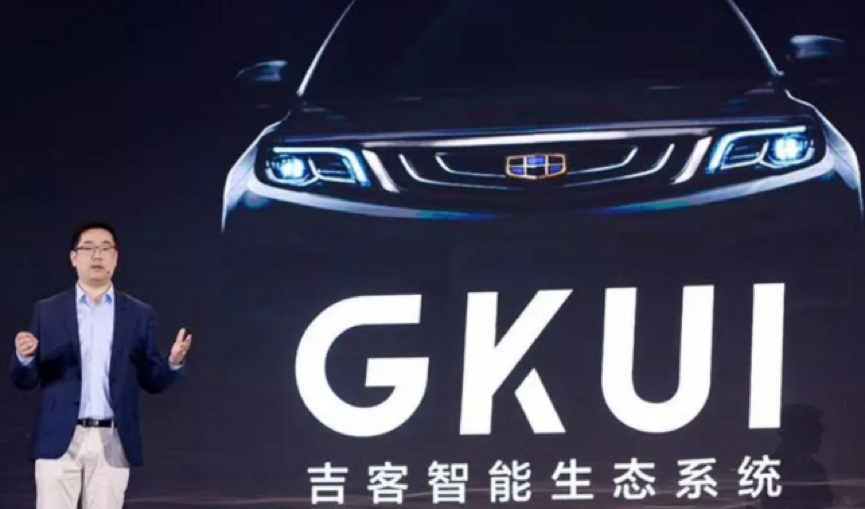中国本土汽车品牌的领跑者吉利汽车今年推出了功能强大、颇具前瞻性的智能生态系统GKUI，接入的就是爱听卓乐独家提供的千万级别的“全to B曲库”，该曲库专为智能车机打造，确保安全合法，杜绝了一切版权风险。经过大半年的合作，爱听和吉利已成功建立起“智能车机+在线音乐服务”的业务模式，为广大吉利车主带来了耳目一新的车载娱乐体验，也为包括音乐版权方、品牌车企、车机厂商等上下游合作伙伴创造了共赢的局面和全新的价值。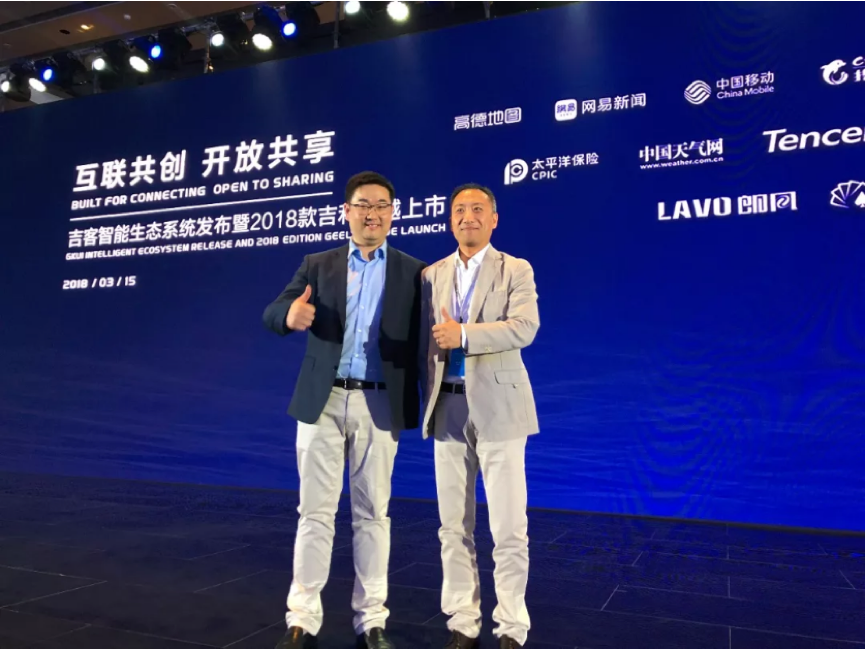吉利研究院副院长沈子瑜 & 爱听卓乐副总裁丁纲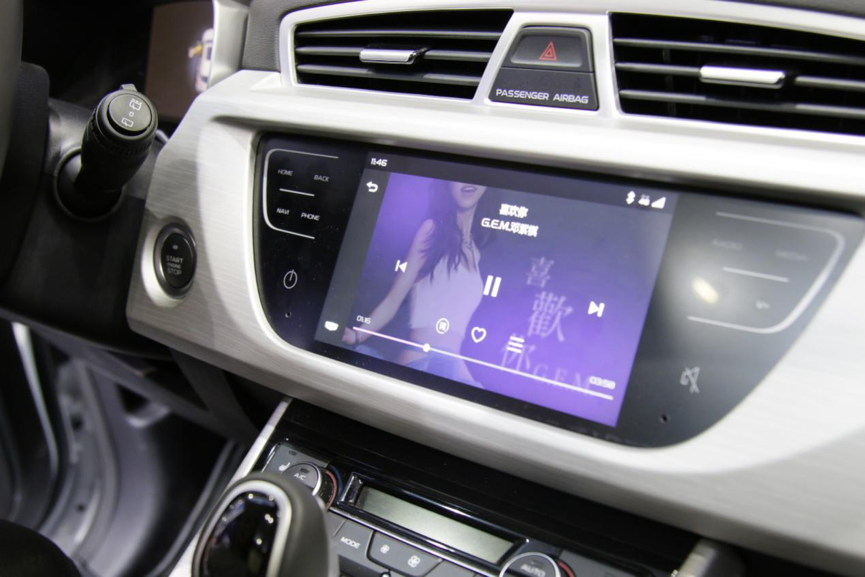搭载了“爱听·车乐云”服务的吉利智能车机系统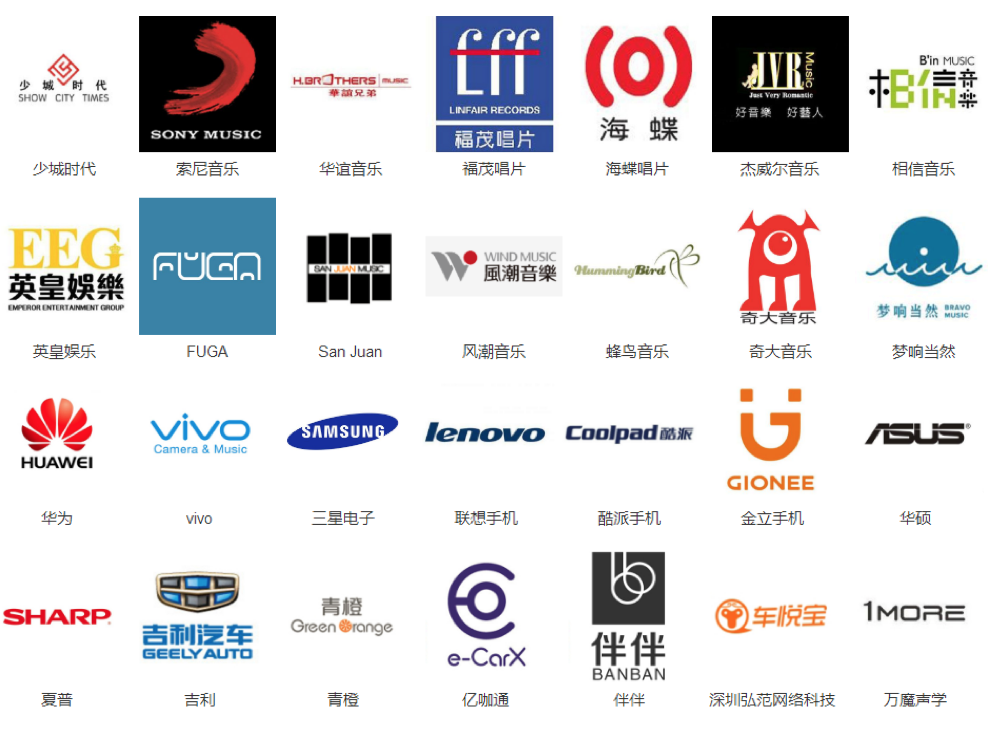除了吉利，爱听卓乐还与众多的整车厂商、方案商、车机厂商缔结了合作关系， 2018下半年将会有越来越多的搭载有爱听车乐云服务的车型投放市场。

相关资讯

|分享

•已阅！握爪
•我手滑为你点赞
•128个赞！
•不明觉厉
•阅后既醉
•有钱！任性
•照片太美，我不敢看
•干货！杠杠的
•高大上
•膜拜中！
•请接受我的膝盖
•猫哥/猫妹么么哒
•神吐槽
•我只笑笑不说话
•我想静静
•窒息
热度排行• 阿斯顿·马丁
• 奥迪
• 阿尔法罗密欧
• ALPINA

• 巴博斯
• 宝骏
• 宝马
• 保时捷
• 北汽制造
• 奔驰
• 奔腾
• 本田
• 比亚迪
• 标致
• 别克
• 宾利
• 布加迪
• 北汽威旺
• 北京
• 北汽绅宝
• 北汽幻速
• 北汽新能源
• 宝沃
• 比速汽车
• 北汽道达

• 昌河
• 长安
• 长城
• 长安商用
• 成功汽车
• 长江EV
• 长安轻车型

• 大众
• 道奇
• 东风
• 东风风神
• 东风小康
• 东南
• DS
• 东风风行
• 东风风度
• 东风风光

• 法拉利
• 菲亚特
• 丰田
• 福迪
• 福特
• 福田汽车
• 福汽启腾
• 风诺

• GMC
• 广汽传祺
• 广汽吉奥
• 观致

• 哈飞
• 海格
• 海马
• 华泰
• 黄海
• 恒天
• 红旗
• 哈弗
• 华颂
• 华凯
• 华泰新能源
• 汉腾汽车

• Jeep
• 江淮
• 江铃
• 捷豹
• 金杯
• 九龙
• 吉利汽车
• 金旅
• 金龙
• 江铃集团轻汽
• 江铃集团新能源
• 君马汽车
• 捷途

• 开瑞
• 凯迪拉克
• 科尼赛克
• 克莱斯勒
• KTM
• 卡威
• 凯翼
• 康迪
• 康迪电动汽车集团

• 猎豹汽车
• 兰博基尼
• 劳斯莱斯
• 雷克萨斯
• 雷诺
• 理念
• 力帆
• 莲花汽车
• 林肯
• 铃木
• 陆风
• 路虎
• 路特斯
• 领志
• 领克
• 零跑汽车

• MG
• MINI
• 马自达
• 玛莎拉蒂
• 迈凯伦
• 摩根

• 纳智捷

• 讴歌
• 欧宝
• 欧朗
• 欧拉
• 欧尚汽车

• 帕加尼

• 奇瑞
• 启辰
• 起亚
• 前途
• 庆铃汽车

• 日产
• 荣威
• 瑞麒汽车
• 如虎
• 瑞驰

• 上汽大通
• smart
• 三菱
• 双环
• 双龙
• 斯巴鲁
• 斯柯达
• 萨博
• 思铭
• 赛麟
• SWM斯威汽车

• TESLA
• 泰卡特
• 腾势

• 威麟
• 威兹曼
• 沃尔沃
• 五菱汽车
• 五十铃
• 潍柴英致
• WEY
• 蔚来
• 威马汽车

• 现代
• 雪佛兰
• 雪铁龙
• 西雅特
• 新特汽车
• 小鹏汽车

• 野马汽车
• 一汽
• 依维柯
• 英菲尼迪
• 永源
• 驭胜

• 中华
• 中兴
• 众泰
• 知豆
• 之诺
• 正道汽车
• A
• B
• C
• D
• E
• F
• G
• H
• I
• J
• K
• L
• M
• N
• O
• P
• Q
• R
• S
• T
• U
• V
• W
• X
• Y
• Z

• 阿斯顿·马丁
• 奥迪
• 阿尔法罗密欧
• ALPINA

• 巴博斯
• 宝骏
• 宝马
• 保时捷
• 北汽制造
• 奔驰
• 奔腾
• 本田
• 比亚迪
• 标致
• 别克
• 宾利
• 布加迪
• 北汽威旺
• 北京
• 北汽绅宝
• 北汽幻速
• 北汽新能源
• 宝沃
• 比速汽车
• 北汽道达

• 昌河
• 长安
• 长城
• 长安商用
• 成功汽车
• 长江EV
• 长安轻车型

• 大众
• 道奇
• 东风
• 东风风神
• 东风小康
• 东南
• DS
• 东风风行
• 东风风度
• 东风风光

• 法拉利
• 菲亚特
• 丰田
• 福迪
• 福特
• 福田汽车
• 福汽启腾
• 风诺

• GMC
• 广汽传祺
• 广汽吉奥
• 观致

• 哈飞
• 海格
• 海马
• 华泰
• 黄海
• 恒天
• 红旗
• 哈弗
• 华颂
• 华凯
• 华泰新能源
• 汉腾汽车

• Jeep
• 江淮
• 江铃
• 捷豹
• 金杯
• 九龙
• 吉利汽车
• 金旅
• 金龙
• 江铃集团轻汽
• 江铃集团新能源
• 君马汽车
• 捷途

• 开瑞
• 凯迪拉克
• 科尼赛克
• 克莱斯勒
• KTM
• 卡威
• 凯翼
• 康迪
• 康迪电动汽车集团

• 猎豹汽车
• 兰博基尼
• 劳斯莱斯
• 雷克萨斯
• 雷诺
• 理念
• 力帆
• 莲花汽车
• 林肯
• 铃木
• 陆风
• 路虎
• 路特斯
• 领志
• 领克
• 零跑汽车

• MG
• MINI
• 马自达
• 玛莎拉蒂
• 迈凯伦
• 摩根

• 纳智捷

• 讴歌
• 欧宝
• 欧朗
• 欧拉
• 欧尚汽车

• 帕加尼

• 奇瑞
• 启辰
• 起亚
• 前途
• 庆铃汽车

• 日产
• 荣威
• 瑞麒汽车
• 如虎
• 瑞驰

• 上汽大通
• smart
• 三菱
• 双环
• 双龙
• 斯巴鲁
• 斯柯达
• 萨博
• 思铭
• 赛麟
• SWM斯威汽车

• TESLA
• 泰卡特
• 腾势

• 威麟
• 威兹曼
• 沃尔沃
• 五菱汽车
• 五十铃
• 潍柴英致
• WEY
• 蔚来
• 威马汽车

• 现代
• 雪佛兰
• 雪铁龙
• 西雅特
• 新特汽车
• 小鹏汽车

• 野马汽车
• 一汽
• 依维柯
• 英菲尼迪
• 永源
• 驭胜

• 中华
• 中兴
• 众泰
• 知豆
• 之诺
• 正道汽车
• A
• B
• C
• D
• E
• F
• G
• H
• I
• J
• K
• L
• M
• N
• O
• P
• Q
• R
• S
• T
• U
• V
• W
• X
• Y
• Z

• 阿斯顿·马丁
• 奥迪
• 阿尔法罗密欧
• ALPINA

• 巴博斯
• 宝骏
• 宝马
• 保时捷
• 北汽制造
• 奔驰
• 奔腾
• 本田
• 比亚迪
• 标致
• 别克
• 宾利
• 布加迪
• 北汽威旺
• 北京
• 北汽绅宝
• 北汽幻速
• 北汽新能源
• 宝沃
• 比速汽车
• 北汽道达

• 昌河
• 长安
• 长城
• 长安商用
• 成功汽车
• 长江EV
• 长安轻车型

• 大众
• 道奇
• 东风
• 东风风神
• 东风小康
• 东南
• DS
• 东风风行
• 东风风度
• 东风风光

• 法拉利
• 菲亚特
• 丰田
• 福迪
• 福特
• 福田汽车
• 福汽启腾
• 风诺

• GMC
• 广汽传祺
• 广汽吉奥
• 观致

• 哈飞
• 海格
• 海马
• 华泰
• 黄海
• 恒天
• 红旗
• 哈弗
• 华颂
• 华凯
• 华泰新能源
• 汉腾汽车

• Jeep
• 江淮
• 江铃
• 捷豹
• 金杯
• 九龙
• 吉利汽车
• 金旅
• 金龙
• 江铃集团轻汽
• 江铃集团新能源
• 君马汽车
• 捷途

• 开瑞
• 凯迪拉克
• 科尼赛克
• 克莱斯勒
• KTM
• 卡威
• 凯翼
• 康迪
• 康迪电动汽车集团

• 猎豹汽车
• 兰博基尼
• 劳斯莱斯
• 雷克萨斯
• 雷诺
• 理念
• 力帆
• 莲花汽车
• 林肯
• 铃木
• 陆风
• 路虎
• 路特斯
• 领志
• 领克
• 零跑汽车

• MG
• MINI
• 马自达
• 玛莎拉蒂
• 迈凯伦
• 摩根

• 纳智捷

• 讴歌
• 欧宝
• 欧朗
• 欧拉
• 欧尚汽车

• 帕加尼

• 奇瑞
• 启辰
• 起亚
• 前途
• 庆铃汽车

• 日产
• 荣威
• 瑞麒汽车
• 如虎
• 瑞驰

• 上汽大通
• smart
• 三菱
• 双环
• 双龙
• 斯巴鲁
• 斯柯达
• 萨博
• 思铭
• 赛麟
• SWM斯威汽车

• TESLA
• 泰卡特
• 腾势

• 威麟
• 威兹曼
• 沃尔沃
• 五菱汽车
• 五十铃
• 潍柴英致
• WEY
• 蔚来
• 威马汽车

• 现代
• 雪佛兰
• 雪铁龙
• 西雅特
• 新特汽车
• 小鹏汽车

• 野马汽车
• 一汽
• 依维柯
• 英菲尼迪
• 永源
• 驭胜

• 中华
• 中兴
• 众泰
• 知豆
• 之诺
• 正道汽车
• A
• B
• C
• D
• E
• F
• G
• H
• I
• J
• K
• L
• M
• N
• O
• P
• Q
• R
• S
• T
• U
• V
• W
• X
• Y
• Z